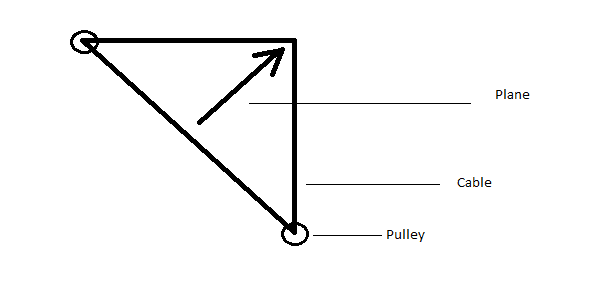# NonLinear calculation of structures

Hello,

I'm a student in mechanical engineering and right now, we're studying non Linear Calculation of Structures. We have a project which consists on evaluating one of the solutions to stop a tourism aeroplane CESSNA 172, like a cable to stop it when it lands.

So we started our study with a Matlab program, and the specifications are to stop the plane within 100m and it's velocity when it's landing is 50m/s. We know that we must have a NonLinear system and study it with the function ODE45 but the problem is that we are not sure is our calculations to have the Differentiel equation are correct or not ! So I'm here to ask u if my problem inspires someone with a method to create the program on Matlab.Thanks very much and if you need more information, I'm here ^^

## Answers and Replies

bigfooted
Gold Member
what differential equation are you solving?

I would implement this in steps with increasing complexity at every step. First, assume it is a simple linear mass-spring-damping system and solve that. It has analytic solutions, so it is easy to compare the numerical result with the analytic result.

At every step (nonlinear spring, nonlinear damping of spring, friction of airplane wheels, drag of airplane) try to find a situation where the equation simplifies and has an analytic solution. There are also known solutions to some nonlinear mass-spring-damping problems, you should be able to verify the implementation of many of the steps.

Finding the best physical model for all the terms in the equation is something else entirely. You can validate that if you have experiments (drag of the plane, nonlinear behavior of the cord...).

Nidum
Science Advisor
Gold Member
Nidum
Science Advisor
Gold Member
For completeness - there are simpler systems using two or three spaced fences of simple netting and nets which are attached to big weights which get dragged .